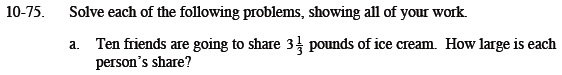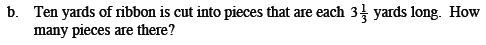### Home > MC1 > Chapter 10 > Lesson 10.2.1 > Problem10-75

10-75.What if there were 20 pounds of ice cream to share?
Each person would get 2 pounds, because 20 pounds divided among 10 people is 2 pounds each.
Try using this same process to find how much ice cream each person gets when there are 3 and one-third pounds of ice cream.

$\frac{3\frac{1}{3}}{10}=\left(\frac{10}{3}\right)\left(\frac{1}{10}\right)=\frac{1}{3}\text{ pound}$$\text{Read this one carefully. In this problem 10 yards are being divided into pieces that are }3\frac{1}{3} \text{ yards long.}$